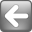## digitalmars.D.bugs - auto bud w/ overloaded function pointers

• BCS (34/34) Nov 26 2005 ``` ```
• ``` ```
``` ```
```BCS <BCS_member pathlink.com> writes: <code> /*** The type of the function pointers and delegates are ambiguous. ***/ void main() { S s; int delegate(int) dlp_i = &s.fn; int delegate() dlp_v = &s.fn; auto dlp_x = &s.fn; dlp_i(1); // works dlp_v(); // works dlp_x(); // what type is dlp_x ?? dlp_x(1); // it depends in the order of fn's in S // same problem with fn ptrs int function(int) fnp_i = &fn; int function() fnp_v = &fn; auto fnp_x = &fn; fnp_i(1); // works fnp_v(); // works fnp_x(); // what type is fnp_x ?? fnp_x(1); // it depends in the order of fn's in S } struct S { int j; // swap these to change the type of dlp_x int fn() { j = 0; return j; } int fn(int i) { j = i; return j; } } // swap these to change the type of fnp_x int fn(int i) { return i; } int fn() { return 0; } </code> Nov 26 2005 <!-- google_ad_client = "pub-5628673096434613"; google_ad_width = 300; google_ad_height = 250; google_ad_format = "300x250_as"; google_ad_type = "text_image"; google_ad_channel ="7651800615"; google_color_border = "336699"; google_color_bg = "FFFFFF"; google_color_link = "0000FF"; google_color_url = "008000"; google_color_text = "000000"; //--> ```
``` ```